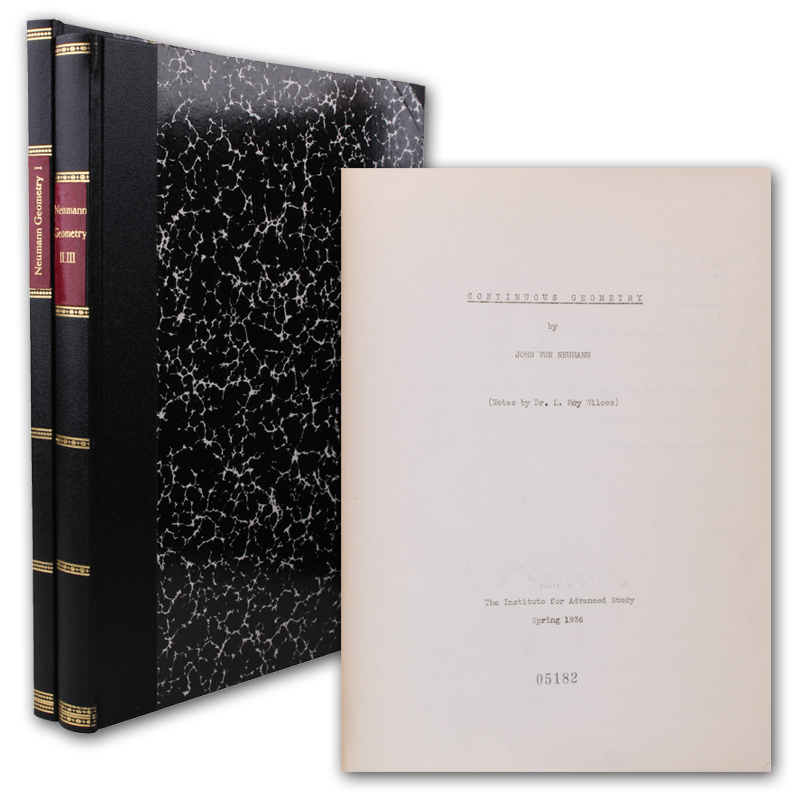# Artikel 112555-01112555-01  Neumann, John von.
Continuous geometry... Notes by L. Roy Wilcox. (27,5 x 21 cm). (2) II, 72 Bll./ (4) 166, 36 S. Moderne Halbleinwandbände im Stil der Zeit. (The Institute for Advanced Study).
(Princeton) und An Arbor, Planographed by Edwards Brother, 1936-37.
Einzige Ausgabe seines seltenen, als Typoskript vervielfältigten Vorlesungsmitschriebs. - "In mathematics, continuous geometry is an analogue of complex projective geometry introduced by von Neumann (1936, 1998), where instead of the dimension of a subspace being in a discrete set 0, 1, ..., n, it can be an element of the unit interval [0,1]. Von Neumann was motivated by his discovery of von Neumann algebras with a dimension function taking a continuous range of dimensions, and the first example of a continuous geometry other than projective space was the projections of the hyperfinite type II factor" (Wikipedia). - "It may be said that the algebraic structure of a factor imposes on the set of corresponding subspaces of H... an order structure similar to that of the subspaces of a usual projective space... Intrigued by this geometric interpretation of his results, von Neumann developed it in a series of papers on 'continuous geometries' and their algebraic satellites, the 'regular rings' (which are to continuous geometries as rings of matrices are to vector spaces). This classification... required great technical skill in the handling of the spectral theory of operators" (DSB). - Titel gestempelt. Die ersten beiden Blätter des 2. Bandes jeweils mit minimaler Eckfehlstelle, sonst sauber und gut erhalten. - DSB 14, 88